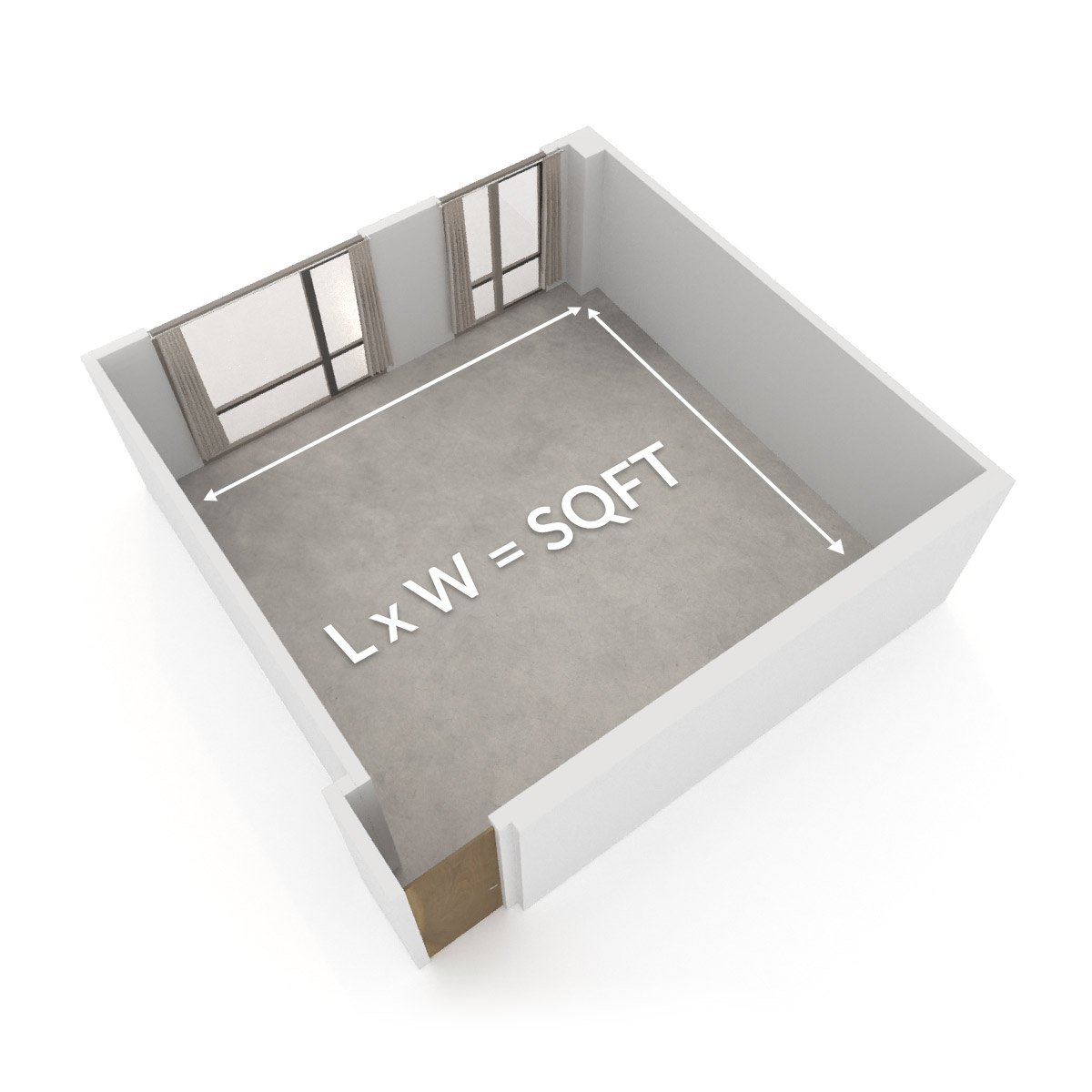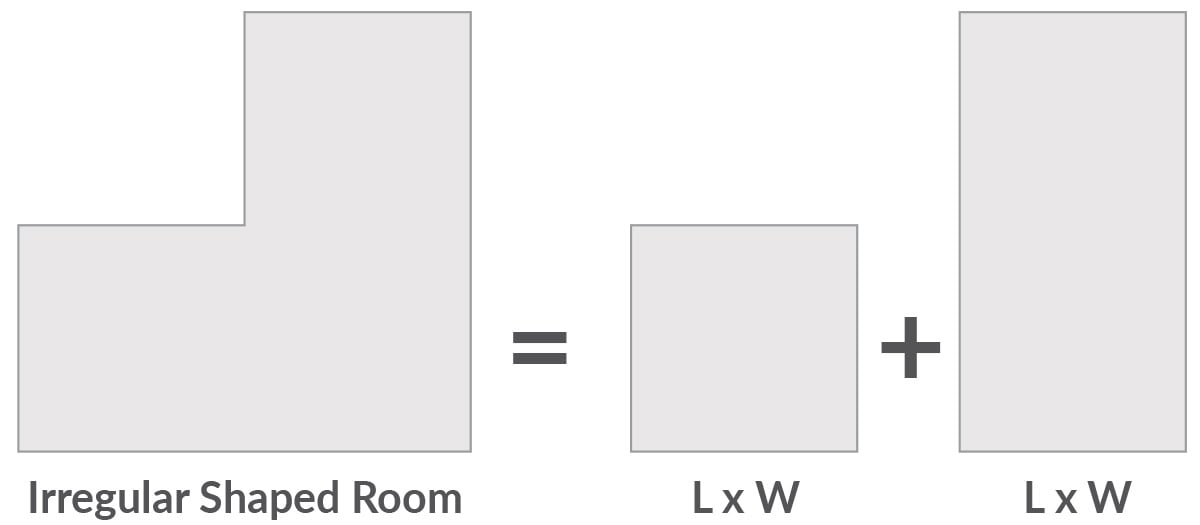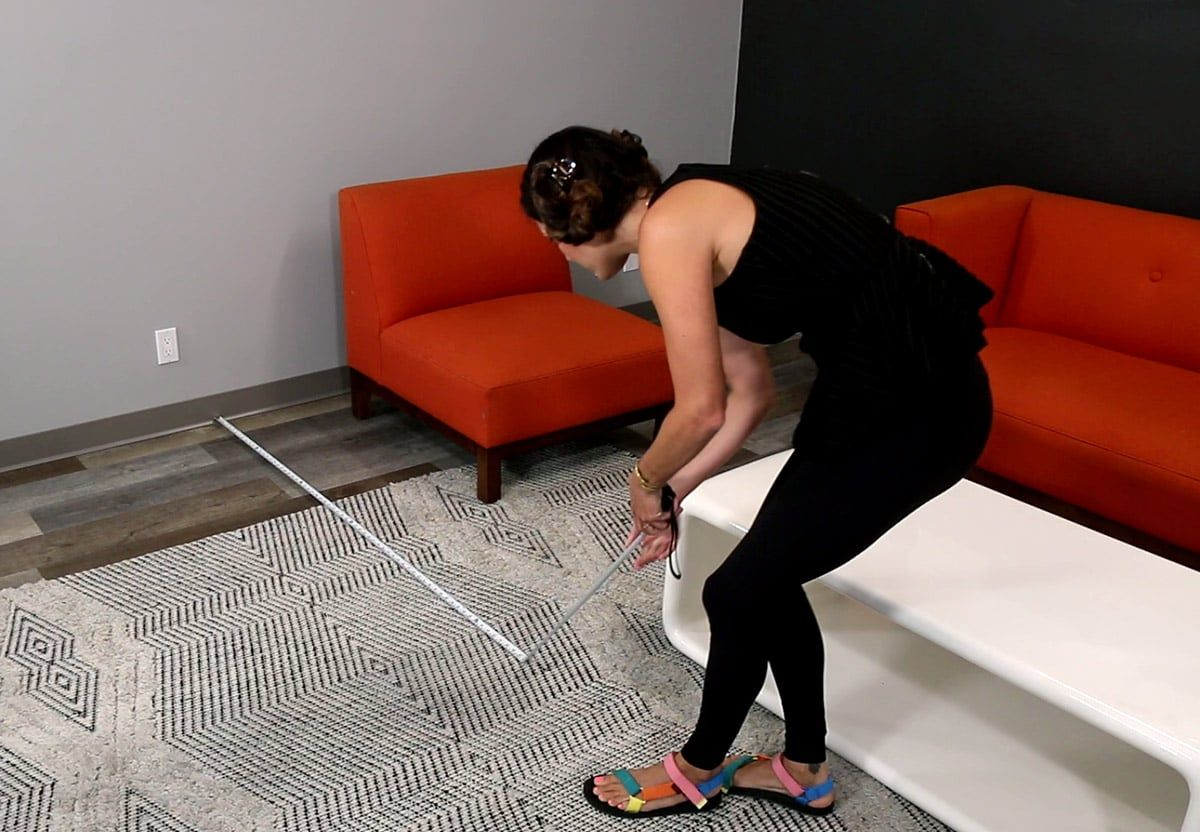0

## HOW TO CALCULATE SQUARE FOOTAGE OF YOUR ROOM EASILY

The first step to many new flooring projects is to understand just how big your space is. Finding the measurements of your room will inform you of how much material you will need to complete the project. To calculate the square footage of your room, you will need a tape measure (or digital measurer) and a pen and notepad (or your phone).

Don't worry if you've never ordered flooring material before, this guide will take you from beginner to measuring expert. We'll start with the square footage formula, then learn to measure irregular shape rooms, and finally, adjust our final project measurements to include excess materials for mistakes and/or waste. The total amount of material you will need will depend on your room size, the material you choose, and your level of experience with installation. Let's start with measuring your room!

## How to Measure the Area

To get started with measuring your area, you will need your tape measure (or digital measurer) and somewhere to record your notes. Feel free to use a pen and paper or the notepad in your phone to log your measurements.

Before measuring, clear away any furniture that would limit your ability to measure your room. A clear space between the opposite walls you're measuring is required so that there are no objects in the way of your measuring tape. To measure the distance between opposite walls, secure one end of your tape measure to the floor against one wall using tape or the assistance of a partner, keep the measuring tape straight until it reaches the next wall. Record the measurement reflected on the measuring tape once it touches the opposing wall in your phone or on paper. Repeat this process to find the distance between the other two opposing walls to determine the room's length and width.

If you are working with a digital measurer, the numbers you'll need to record will be reflected on the device's screen rather than the tape itself.
When using a tape measure, ensure the tape is kept straight. The tape should not be bent, nor should you measure around objects.## How to Calculate Square Footage

Once you've got the measurements for the distances between your two sets of walls, you know the length and width of your room. These dimensions are essential to calculate the square footage of your room.

To find the area of a rectangle or the square footage of your room, we will multiply the length by the width.

Length x Width = Square Footage

For example, say you've measured the distance between the walls of your room and discovered that there are 12 feet between the first two walls and 12 feet between the second two walls. In this case, your length and width would be 12 feet by 12 feet. You will multiply the 12 foot width by the 12 foot length to find the square footage of your room which is 144 square feet.

12 ft x 12 ft = 144 ft²

### Converting Inches to Feet

When room measurements are not a whole foot, how do you convert inches to feet? Since there are 12 inches in 1 foot, to convert inches to feet, simply divide the inches by 12.

For example, if your room measured at 10 feet 3 inches wide, you could convert that measurement to feet by dividing 3 inches by 12 which is .25, and adding it to the 10 feet.

3 inches / 12 = .25 feet

Therefore, the width of the room is 10.25 feet.

If the room you're measuring is a square or rectangle, feel free to skip over the irregular shapes section to thesquare footage calculator.

## How to Calculate Square Feet of Irregular Shaped Rooms

It is common for rooms to not be a perfect rectangle or square. In these cases, we will include additional steps to calculate the square footage of irregular shaped rooms. The additional step will use the same square footage formula we've already reviewed and add the multiplied length and width of the irregular shape.

Length x Width = Square Footage of Main Section

Length x Width = Square Footage of Small Section

Square Footage of Main Section + Square Footage of Small Section

= Total Square Footage of the Irregular RoomFor example, the main section of a room measures out to 12 feet by 12 feet, and an additional space of the room measures out to 5 feet by 7 feet. To find the square footage of this irregular-shaped room, we will find the square footage of the main section and the small section and then add them together.

Square Footage of Main Section

12 ft x 12 ft = 144 ft2

Square Footage of Small Section

5 ft x 7 ft = 35 ft2

Total Square Footage of the Irregular Room

144 ft2 + 35 ft2 = 179 ft2## Square Footage Calculator

Once you have the dimensions of your room recorded, use the calculator below to find the square footage of your room. Use the fields labeled length and width to input your measurements and click 'Calculate'.

For non-rectangle, irregularly shaped rooms press the 'Add Space' button to have the option to add additional lengths and widths to your square footage calculation.

The next step will help you determine how much flooring material you'll need to purchase for your flooring project.

Example:12.25 (12' 3")

## How Much Flooring Do I Need?

Once you have found the length and width of your room and calculated its square footage, you'll need to adjust for the probable waste. This is to compensate for mistakes and other unforeseeable accidents as you complete your project. The amount of excess material you'll need in addition to the room's square footage will depend on your project's material and your level of installation experience. CALI recommends an additional 5% of material for vinyl flooring projects and an additional 10% for engineered or solid hardwood flooring projects. That said, you may be wondering, "how much flooring do I need?".

Let's continue with the examples above. If we have determined that the square footage of a room is 179 ft2, then we need to add a percentage of excess material in order to find the total material to order. The process looks like this:

Square Footage of Room x Percent Excess Material = Excess Material Needed

Excess Material Needed + Square Footage of Room = Total Square Footage to Order

The total amount of material we will need for a vinyl flooring project in a 179 ft2 room is 188 ft2 (rounded to the nearest square foot). To find this, we'll convert 5% to a decimal and use the formula introduced above.

Amount of Excess Material Needed

179 ft2 * .05 = 8.95 ft2

Total Materials to Order

179 ft2 + 8.95 ft2 = 187.95 ft2

To find the total amount of flooring material we'll need, use the calculator below. Enter your room's square footage and the percentage of extra material you anticipate needing and click 'Calculate'.

* Rounded up to the nearest foot

If you're still unsure about the amount of excess material you may need, you can call the flooring experts at CALI at 1(888) 788-2254 for advice.

## What's Next?

Throughout this guide we've used the length x width formula to calculate the square footage of a room for flooring, we have discussed irregularly shaped rooms (including circular and triangular spaces), and determined how much extra material you'll need on top of your room's square footage to make up for mistakes and plank adjustments.

So, now that you know the total square footage of material you'll want to order for your project, what's next? If you haven't selected a material for your room yet, explore our guide below to learn about choosing a material that meets your needs.